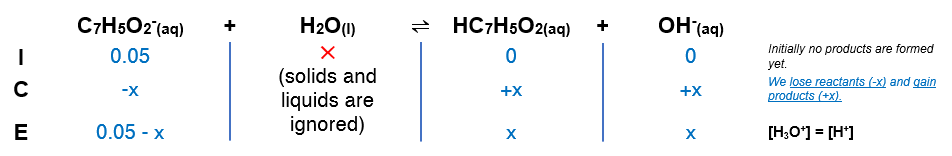# Problem: Calculate the pH at the halfway point and at the equivalence point for each of the following titrations.a. 100.0 mL of 0.10 M HC7H5O2 (Ka = 6.4 x 10-5) titrated by 0.10 M NaOH

###### FREE Expert Solution

a. at half equivalence point

• At half equivalence point for weak species + strong species, the moles of each species are equal
• Therefore, pH is equal to the pKa

pH = - log (6.4x10-5) = 4.19

b. at equivalence point

• The pH will be determined by the c.base (C7H5O2-) produced by the reaction.
• If both species (weak and strong) have the same concentration, the concentration of the conjugate species is half of the concentration:

0.1 M → 0.05 M

Calculating the pH:

(1) Setup an ICE chart:(2) Setup Kb expression

$\overline{){{\mathbf{K}}}_{{\mathbf{b}}}{\mathbf{=}}\frac{\mathbf{products}}{\mathbf{reactants}}{\mathbf{=}}\frac{\mathbf{\left[}{\mathbf{OH}}^{\mathbf{-}}\mathbf{\right]}\mathbf{\left[}{\mathbf{HC}}_{\mathbf{7}}{\mathbf{H}}_{\mathbf{5}}{\mathbf{O}}_{\mathbf{2}}\mathbf{\right]}}{\mathbf{\left[}{\mathbf{C}}_{\mathbf{7}}{\mathbf{H}}_{\mathbf{5}}{{\mathbf{O}}_{\mathbf{2}}}^{\mathbf{-}}\mathbf{\right]}}}$

Solving for x = [OH-]###### Problem Details

Calculate the pH at the halfway point and at the equivalence point for each of the following titrations.

a. 100.0 mL of 0.10 M HC7H5O2 (Ka = 6.4 x 10-5) titrated by 0.10 M NaOH